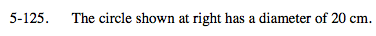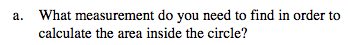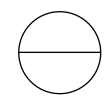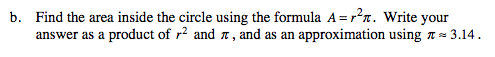### Home > MC2 > Chapter 5 > Lesson 5.3.4 > Problem5-125

5-125.
1. The circle shown below has a diameter of 20 cm. Homework Help ✎

1. What measurement do you need to find in order to calculate the area inside the circle?2. Find the area inside the circle using the formula A = r2 π. Write your answer as a product of r2 and π, and as an approximation using π ≈ 3.14.Recall the equation for area from the Math Notes box of Lesson 5.3.4.

A = r2 π

What measurement is missing in this equation?